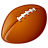# Densifiy a Circle

573
7
12-15-2017 12:51 PMby
Occasional Contributor III

I cannot get any of the density functions to work correctly on a true circle.  I simple want to draw a circle using the Circle create feature tool, then turn that circle into many points.  I want to do this, because i have found that when clipping and getting the difference of two circles, it works better if they are densified.  The problem is, is that everytime I try any of the 4 densify functions in Pro SDK, the circles turn into Oblong ovals.  I need them to preserve their circular shape, but have many vertices.

In ArcObjects I simply did this

``newPolygon.Densify(.0001, .0001);‍‍``

But in ArcGIS Pro, i cant get anything to work quite like that?  They all turn into ovals, not the true circle

``````densifyPolygon = GeometryEngine.Instance.GeodeticDensifyByDeviation(densifyPolygon,.0001,LinearUnit.Feet,GeodeticCurveType.Geodesic);
densifyPolygon = GeometryEngine.Instance.DensifyByLength(densifyPolygon, .01);
densifyPolygon = GeometryEngine.Instance.DensifyByDeviation(densifyPolygon, .0001);

etc...

‍‍‍‍‍‍‍‍‍‍‍‍‍‍‍‍‍‍‍‍‍‍‍‍‍‍‍``````
Tags (2)
1 Solution

Accepted Solutionsby
Occasional Contributor III

Thank you for all of your help.  I think I have the solution now.  It was a combination of my projection before and after the densify was executed.  Additionally, using 1m distance was more than sufficient for my needs.  I projected my item to WebMercator, then densified, then projected back to my original Spatial Reference.  I need to still check that the shape was correctly preserved, but this seemed to do the trick

``````SpatialReference previousSR = densifyPolygon.SpatialReference;

//Need to get to WebMercator in order to get the circle the same
densifyPolygon = GeometryEngine.Instance.Project(densifyPolygon, SpatialReferences.WebMercator);
densifyPolygon = GeometryEngine.Instance.DensifyByLength(densifyPolygon, 1);
densifyPolygon = GeometryEngine.Instance.Project(densifyPolygon, previousSR);

_dataInspector.Shape = densifyPolygon;‍‍‍‍‍‍‍‍``````
7 RepliesbyMVP Esteemed Contributor

What projection are you using and how large are these circles?by
Occasional Contributor III

These circles represent an irrigation system in an agriculture field. So around 200-400m in diameter.  The projection is UTM with the meridian centered for that area where the fields are at to best preserve distance.  Is there a particular projection that would be best for this that would preserve the circle during the densify procedure?byMVP Esteemed Contributor

a densification of 0.001 would represent 1 millimeter if your file is indeed in a utm projection... perhaps specify your densification in units appropriate to the circumference of the circle.  most arc* based circles use an n-gon of 360 points to represent a circle.  You can calculate your current point spacing from the circumference/360 then you can space appropriately

Now... if the file is actually in decimal degrees... project it to UTM first since guessing spacing from arc-seconds is less than desirableby
Occasional Contributor III

My users use the Circle tool from the editing tool bar to draw the circle via heads up digitizing.  But I need this circle to be converted from the 2 points of a circle, to a densified circle~ of points.

My problem can be recreated by taking the circle created using the out of box circle edit tool, then in the onRowCreated event try to densify it.  I always get the oblong circle or oval.byMVP Esteemed Contributor

The oblongness could be a visual artifact, i.e., the shape isn't actually oblong but appears that way on the screen, or it could be the original circle isn't true because it is based on 2 points but can only be displayed as a true circle.

I would check the areas, perimeters, and coordinates of where the two shapes cross axes.  That will at least help inform whether the oblongness is purely visual or whether the shapes are changing during densification.

Also, 1 millimeter vertices is crazy dense.  What if you densify at 1 decimeter, 1 meter, etc...  Does the oblongness get less or more?byMVP Esteemed Contributor

If the circle is oblong, then I suspect that the map viewer is in geographic coordinates and not in a projected coordinate system like UTMby
Occasional Contributor III

Thank you for all of your help.  I think I have the solution now.  It was a combination of my projection before and after the densify was executed.  Additionally, using 1m distance was more than sufficient for my needs.  I projected my item to WebMercator, then densified, then projected back to my original Spatial Reference.  I need to still check that the shape was correctly preserved, but this seemed to do the trick

``````SpatialReference previousSR = densifyPolygon.SpatialReference;

//Need to get to WebMercator in order to get the circle the same
densifyPolygon = GeometryEngine.Instance.Project(densifyPolygon, SpatialReferences.WebMercator);
densifyPolygon = GeometryEngine.Instance.DensifyByLength(densifyPolygon, 1);
densifyPolygon = GeometryEngine.Instance.Project(densifyPolygon, previousSR);

_dataInspector.Shape = densifyPolygon;‍‍‍‍‍‍‍‍``````